## Sunday, 5 October 2014

### EXPLANATION ON MICROSCOPE BY USING RAY DIAGRAM AND EXAMPLE

A basic microscope is made up of two converging lenses. One reason for using two lenses rather than just one is that it's easier to get higher magnification. If you want an overall magnification of 35, for instance, you can use one lens to magnify by a factor of 5, and the second by a factor of 7. This is generally easier to do than to get magnification by a factor of 35 out of a single lens.
A microscope arrangement is shown below, along with the ray diagram showing how the first lens creates a real image. This image is the object for the second lens, and the image created by the second lens is the one you'd see when you looked through the microscope.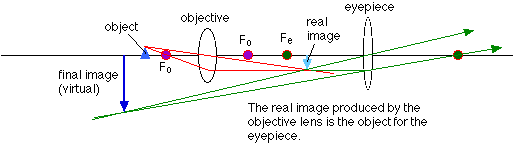Note that the final image is virtual, and is inverted compared to the original object. This is true for many types of microscopes and telescopes, that the image produced is inverted compared to the object.

### An example using the microscope

Let's use the ray diagram for the microscope and work out a numerical example. The parameters we need to specify are: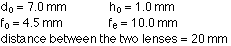To work out the image distance for the image formed by the objective lens, use the lens equation, rearranged to: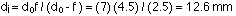The magnification of the image in the objective lens is: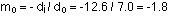So the height of the image is -1.8 x 1.0 = -1.8 mm.
This image is the object for the second lens, and the object distance has to be calculated:The image, virtual in this case, is located at a distance of:The magnification for the eyepiece is: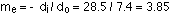So the height of the final image is -1.8 mm x 3.85 = -6.9 mm.
The overall magnification of the two lens system is: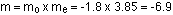This is equal to the final height divided by the height of the object, as it should be. Note that, applying the sign conventions, the final image is virtual, and inverted compared to the object. This is consistent with the ray diagram.